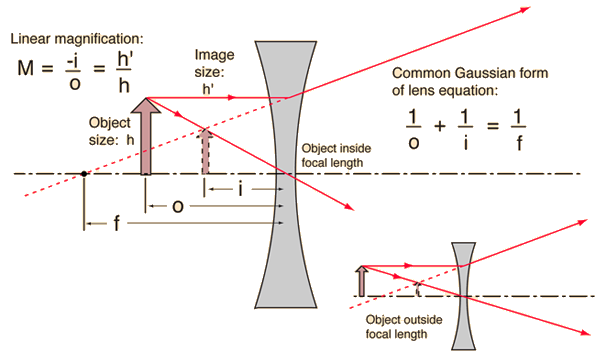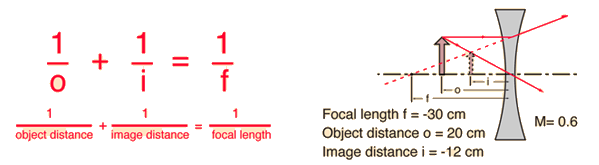# Virtual Image Formation

Diverging lenses form reduced, erect, virtual images. Using the common form of the lens equation, f, P and i are negative quantities.Enter data below, then click on the quantity you wish to calculate in the active formula above.
For a lens of focal length f = cm,
corresponding to lens power P = diopters,
an object distance of o = cm
will produce an image at i = cm.
The linear magnification will be M = .

If a negative focal length is entered to agree with the illustration, then the image is a virtual image on the same side of the lens as the object and will give a negative image distance. If a calculation yields a positive focal length, then the lens is a converging lens rather than the diverging lens in the illustration. The lens equation can be used to calculate the image distance for either real or virtual images and for either positive on negative lenses. The linear magnification relationship allows you to predict the size of the image.

 Other ways to form virtual images
Index

Lens concepts

 HyperPhysics***** Light and Vision R Nave
Go Back